# Roots of Quadratic Equations in C++

## Roots of a Quadratic Equation in C++:

In this article, we will write a program for finding the Roots of a Quadratic Equation in C++ with Examples. Please read our previous article where we discussed the Sum of First N Natural Numbers in C++ with Examples.

##### What is a Quadratic Equation?

Let us understand what is a quadratic equation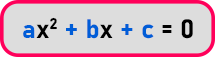It is an equation of this form that is a polynomial of the form of x2, x, and x0. The highest degree is 2 i.e. x2. So, a polynomial of degree 2 is a quadratic expression and when the expression is equal to 0 then it is a quadratic equation.

Then the coefficients of the equation are used to find the roots of the equation means what are the possible values of x. We got the possible values of x if we know the value of a, b and c. So, roots can be known by using the below formula: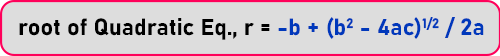So actually, we will know for what values of x this equation will be equal to 0. The values at which the whole quadratic equation is equal to zero are known as the roots of the quadratic equation. Now for this, we will write a program that will take the input, and find out the roots and give the output.

So, let us see what is input? Input is the value of coefficients i.e. a, b, and c. these three variables are input. And the root is the output. So let’s first draw the flowchart:

##### Roots of Quadratic Equation Flowchart:

First, we have to start the flowchart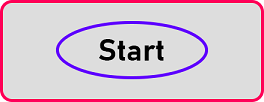Then we have to take input from the user. But what is the input? Input is the value of coefficients a, b and c. So, inside this input/output box we give a message ‘Enter coefficients:’ or instead of coefficients we will print ‘Enter a, b and c’. Now we will read a, b and c so I should take the values.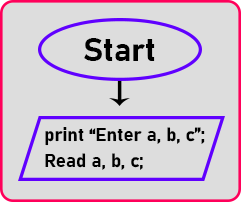Now, next is the process part. Actually, we get two roots because one is with the addition and one is with subtraction, so r1 is the first root and r2 is the second root.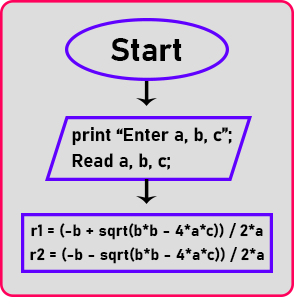Here I got two roots now I have to give the output that is the result we will print a message that ‘roots are’ then r1 and r2.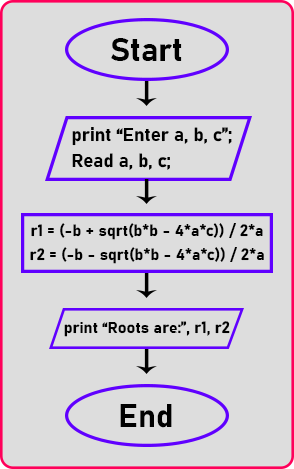Here is the end of the flowchart. Now we will write a C++ program. So let us convert this flowchart into a C++ program.

##### Roots of Quadratic Equation Code in C++ Language:
```#include <iostream>
#include <math.h>
using namespace std;
int main ()
{
float a, b, c, r1, r2;
cout << "Enter a, b, c: ";
cin >> a >> b >> c;
r1 = (-b + sqrt (b * b - 4 * a * c)) / 2 * a;
r2 = (-b - sqrt (b * b - 4 * a * c)) / 2 * a;
cout << "Roots are: " << r1 << " " << r2;
return 0;
}
```
###### Output: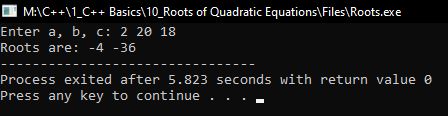In the next article, I am going to discuss Programming Exercises in C++ with Examples. Here, in this article, I try to explain the Roots of Quadratic Equations in C++ with Examples and I hope you enjoy this Roots of Quadratic Equations in C++ with Examples article.